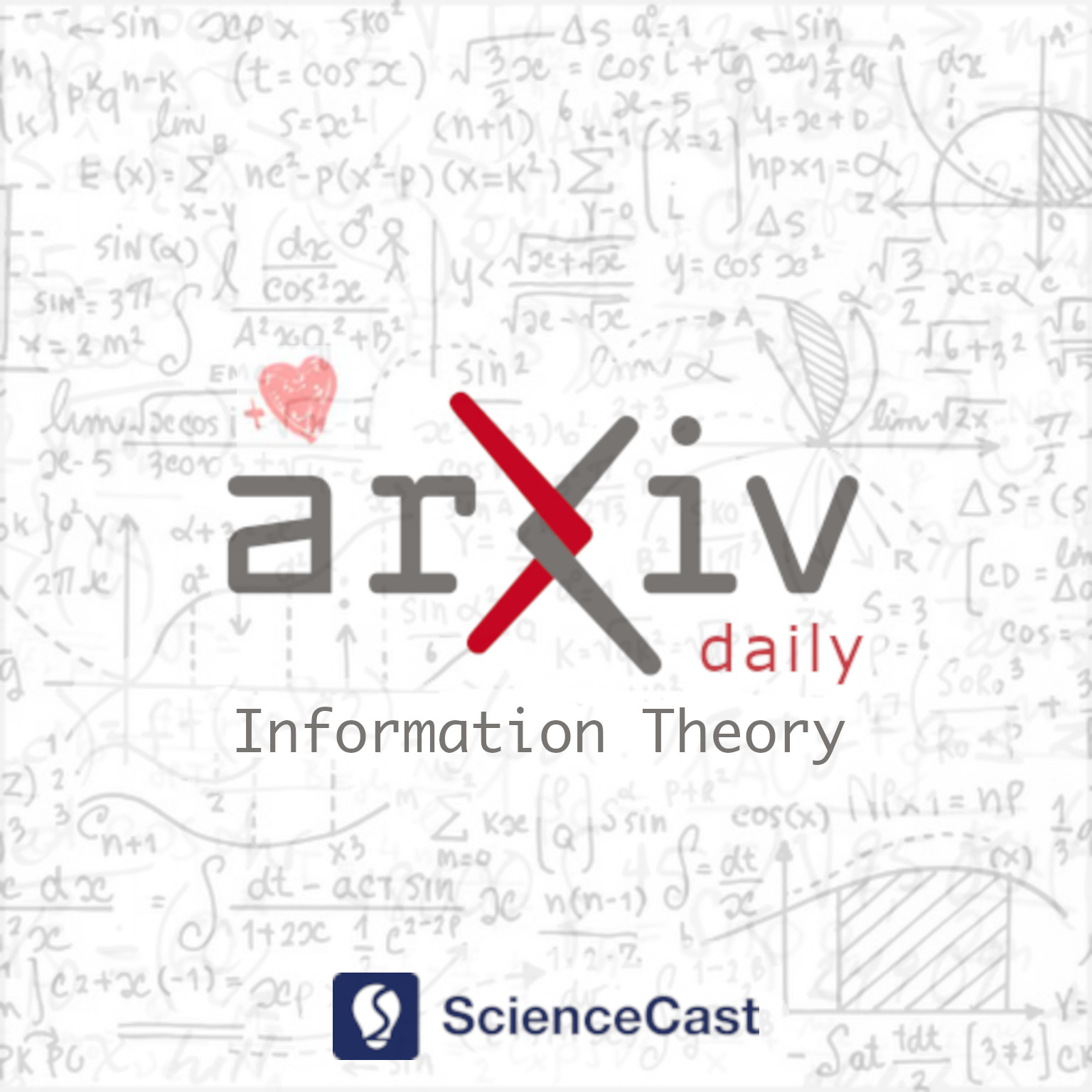##### 1.Quantum Cross Subspace Alignment Codes via the $N$-sum Box Abstraction

Authors:Yuxiang Lu, Syed Ali Jafar

Abstract: Cross-subspace alignment (CSA) codes are used in various private information retrieval (PIR) schemes (e.g., with secure storage) and in secure distributed batch matrix multiplication (SDBMM). Using a recently developed $N$-sum box abstraction of a quantum multiple-access channel (QMAC), we translate CSA schemes over classical multiple-access channels into efficient quantum CSA schemes over a QMAC, achieving maximal superdense coding gain. Because of the $N$-sum box abstraction, the underlying problem of coding to exploit quantum entanglements for CSA schemes, becomes conceptually equivalent to that of designing a channel matrix for a MIMO MAC subject to given structural constraints imposed by the $N$-sum box abstraction, such that the resulting MIMO MAC is able to implement the functionality of a CSA scheme (encoding/decoding) over-the-air. Applications include Quantum PIR with secure and MDS-coded storage, as well as Quantum SDBMM.

##### 2.Polynomial time attack on high rate random alternant codes

Authors:Magali Bardet, Rocco Mora, Jean-Pierre Tillich

Abstract: A long standing open question is whether the distinguisher of high rate alternant codes or Goppa codes \cite{FGOPT11} can be turned into an algorithm recovering the algebraic structure of such codes from the mere knowledge of an arbitrary generator matrix of it. This would allow to break the McEliece scheme as soon as the code rate is large enough and would break all instances of the CFS signature scheme. We give for the first time a positive answer for this problem when the code is {\em a generic alternant code} and when the code field size $q$ is small : $q \in \{2,3\}$ and for {\em all} regime of other parameters for which the aforementioned distinguisher works. This breakthrough has been obtained by two different ingredients : (i) a way of using code shortening and the component-wise product of codes to derive from the original alternant code a sequence of alternant codes of decreasing degree up to getting an alternant code of degree $3$ (with a multiplier and support related to those of the original alternant code); (ii) an original Gr\"obner basis approach which takes into account the non standard constraints on the multiplier and support of an alternant code which recovers in polynomial time the relevant algebraic structure of an alternant code of degree $3$ from the mere knowledge of a basis for it.

##### 3.Channel Orthogonalization with Reconfigurable Surfaces

Authors:Juan Vidal Alegria, Fredrik Rusek

Abstract: Orthogonal multi-user multiple-input multiple-output (MU-MIMO) channels allow for optimum performance with simplified precoding/equalization, and they achieve maximum multiplexing gain which is shared fairly among users. Reconfigurable intelligent surface (RIS) constitutes a promising cost-efficient solution to improve the wireless channel, since they consist of passive reflecting elements able to adjust the phases of the incoming waves. However, it is still widely unclear how these surfaces can improve spatial-multiplexing. In fact, the common RIS model cannot achieve perfect orthogonalization of MU-MIMO channels with a reasonable number of elements. Furthermore, efficient channel estimation algorithms for RIS, which are key for taking advantage of its benefits, are still a matter of research. We study two types of reconfigurable surfaces (RSs), namely amplitude-reconfigurable intelligent surface (ARIS) and fully-reconfigurable intelligent surface (FRIS), with extended capabilities over RIS. We show how these RSs allow for perfect channel orthogonalization, and, by minimizing the applied power, we show that they can potentially be implemented without the need of amplification. We also present an efficient channel estimation method for each of them that allows the base station (BS) to select the desired propagation channel.

##### 4.Estimation of Interference Correlation in mmWave Cellular Systems

Authors:Stefano Tomasin, Raphael Hasler, Antonia M. Tulino, Matilde Sánchez-Fernández

Abstract: We consider a cellular network, where the uplink transmissions to a base station (BS) are interferenced by other devices, a condition that may occur, e.g., in cell-free networks or when using non-orthogonal multiple access (NOMA) techniques. Assuming that the BS treats this interference as additional noise, we focus on the problem of estimating the interference correlation matrix from received signal samples. We consider a BS equipped with multiple antennas and operating in the millimeter-wave (mmWave) bands and propose techniques exploiting the fact that channels comprise only a few reflections at these frequencies. This yields a specific structure of the interference correlation matrix that can be decomposed into three matrices, two rectangular depending on the angle of arrival (AoA) of the interference and the third square with smaller dimensions. We resort to gridless approaches to estimate the AoAs and then project the least square estimate of the interference correlation matrix into a subspace with a smaller dimension, thus reducing the estimation error. Moreover, we derive two simplified estimators, still based on the gridless angle estimation that turns out to be convenient when estimating the interference over a larger number of samples.

##### 5.Randomness Requirements for Three-Secret Sharing

Authors:Hari Krishnan P. Anilkumar, Aayush Rajesh, Varun Narayanan, Manoj M. Prabhakaran, Vinod M. Prabhakaran

Abstract: We study a secret sharing problem with three secrets where the secrets are allowed to be related to each other, i.e., only certain combinations of the three secrets are permitted. The dealer produces three shares such that every pair of shares reveals a unique secret and reveals nothing about the other two secrets, other than what can be inferred from the revealed secret. For the case of binary secrets, we exactly determine the minimum amount of randomness required by the dealer, for each possible set of permitted combinations. Our characterization is based on new lower and upper bounds.